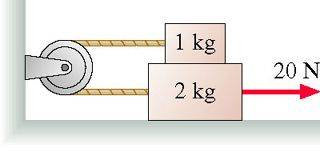# Kinetic Friction problem

mvpshaq32

## Homework Statement

The lower block in the figure is pulled on by a rope with a tension force of 20 N. The coefficient of kinetic friction between the lower block and the surface is 0.30. The coefficient of kinetic friction between the lower block and the upper block is also 0.30.Find the acceleration of the 2.0kg block

## The Attempt at a Solution

I set m1a= T - (0.3)m1g and m2a= 20 - T - 0.3m1+2g. After which I added them and isolating a, giving me a=(20 - 0.3m1+2g - 0.3m1g)/(m1+m2), which gave me the answer 2.75, but apparently it is not correct.

Never mind, I solved it. I forgot the friction between block 1 and 2 for movement of block 2

Last edited:

## Answers and Replies

Vipho
m1a= T - (0.3)m1g
m2a= 20 - T - 0.3m1+2g-0.3m1g

==>a=[20-(m1+m2)g*0.3-2*0.3*m1g]/(m1+m2)=5/3 (ms^-2)

is it right?

mvpshaq32
Yep, you got the equation set up right, but the answer is 1.77, not 5/3